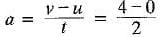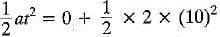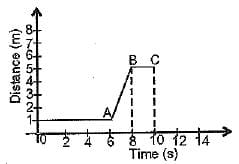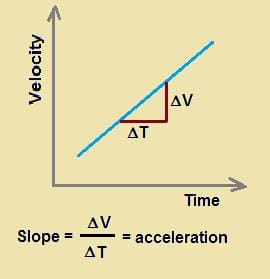Test: Motion- 2

# Test: Motion- 2

Test Description

## 10 Questions MCQ Test Science Class 9 | Test: Motion- 2

Test: Motion- 2 for Class 9 2023 is part of Science Class 9 preparation. The Test: Motion- 2 questions and answers have been prepared according to the Class 9 exam syllabus.The Test: Motion- 2 MCQs are made for Class 9 2023 Exam. Find important definitions, questions, notes, meanings, examples, exercises, MCQs and online tests for Test: Motion- 2 below.
Solutions of Test: Motion- 2 questions in English are available as part of our Science Class 9 for Class 9 & Test: Motion- 2 solutions in Hindi for Science Class 9 course. Download more important topics, notes, lectures and mock test series for Class 9 Exam by signing up for free. Attempt Test: Motion- 2 | 10 questions in 10 minutes | Mock test for Class 9 preparation | Free important questions MCQ to study Science Class 9 for Class 9 Exam | Download free PDF with solutions
 1 Crore+ students have signed up on EduRev. Have you?
Test: Motion- 2 - Question 1

### Equations of motion can be used for a body having______.

Detailed Solution for Test: Motion- 2 - Question 1

The equations of motion are applicable only when the body moves with uniform acceleration. A body under constant acceleration is uniformly accelerated motion.

Test: Motion- 2 - Question 2

### The magnitude of the displacement of an object is always______.

Detailed Solution for Test: Motion- 2 - Question 2
• Displacement is the shortest straight line distance between the initial and final position. This means that there doesn't exist any other path which is shorter than it.
• If the object travels with that shortest straight-line path then the magnitude of distance and displacement would be the same.
• If the object doesn't do so and travels with any other paths then as the other path is not the shortest path, so it will be larger than that of displacement. So if the object travels with this path then the magnitude of displacement will be smaller than the distance.

Thus, the magnitude of displacement may be equal to or smaller than that of distance.

Test: Motion- 2 - Question 3

### For a body starting from rest, the displacement in 10 seconds, when it acquires 4 ms-1 in 2 seconds is______.

Detailed Solution for Test: Motion- 2 - Question 3

v = u + at
or= 2m/s2
s = ut += 100 m

Test: Motion- 2 - Question 4

Retardation of a body is expressed in________.

Detailed Solution for Test: Motion- 2 - Question 4
• Retardation, actually, is acceleration that acts opposite to the direction of motion of an object.
• So, under retardation, an object accelerates away from its direction of motion, so its velocity in that direction decreases.
• The SI unit of retardation, which is just an oppositely directed acceleration, is m/s2.
Test: Motion- 2 - Question 5

The v-t graph for the x-t graph given here is best drawn asTest: Motion- 2 - Question 6

On a velocity-time graph, a horizontal straight line corresponds to motion at_______.

Detailed Solution for Test: Motion- 2 - Question 6

It is horizontal so, angle is zero and also acceleration is zero. Hence constant velocity.

Test: Motion- 2 - Question 7

For a uniformly accelerated body with initial and final velocities as u and v ms-1, the average velocity is _______.

Detailed Solution for Test: Motion- 2 - Question 7

Average velocity of an object is equal to its final velocity plus initial velocity, divided by 2.
Therefore, the answer is, (u + v) / 2

Test: Motion- 2 - Question 8

Which of the following graphs show that the body is at rest?

Detailed Solution for Test: Motion- 2 - Question 8

In figure A, we can see that the object continues to remain at a fixed place while the time keeps running. So, the object is at rest, because the object does not change its position with time.

Test: Motion- 2 - Question 9

The area below v - t graph is a measure of_______.

Detailed Solution for Test: Motion- 2 - Question 9

"Displacement" is the quantity that is measured by the area occupied under the velocity-time graph. Moving something or someone from its place or position is called displacement.

Test: Motion- 2 - Question 10

The slope of velocity-time graph gives_______.

Detailed Solution for Test: Motion- 2 - Question 10The slope of a velocity-time graph represents the acceleration of the object. So, the value of the slope at a particular time represents the acceleration of the object at that instant.

## Science Class 9

115 videos|302 docs|163 tests
 Use Code STAYHOME200 and get INR 200 additional OFF Use Coupon Code
Information about Test: Motion- 2 Page
In this test you can find the Exam questions for Test: Motion- 2 solved & explained in the simplest way possible. Besides giving Questions and answers for Test: Motion- 2, EduRev gives you an ample number of Online tests for practice

## Science Class 9

115 videos|302 docs|163 tests